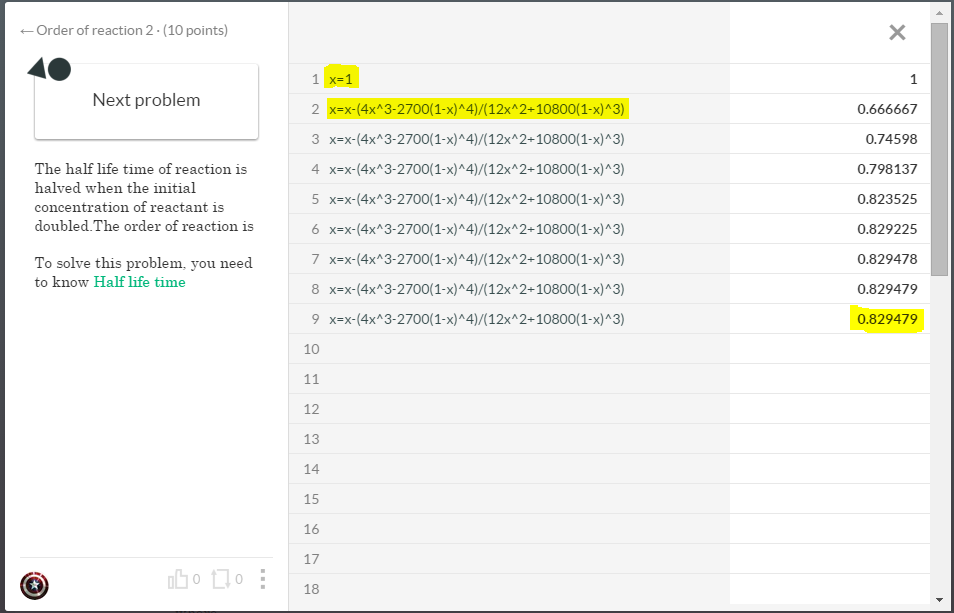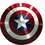# Have you ever used Scratch pad like this?

Find the root of following equation.

$4y^3-2700(1-y)^4=0$

Above equation can be solved using newton raphson iterative method with initial approximation to be unity.

1) First Let $f(y)=4y^3-2700(1-y)^4$ We have to find root of above function.

2)Find derivative of $f(y)$. Here,$f^{'}(y)=12y^2+10800(1-y)^3$

3)Find $y_n$ using newton raphson method $y_0=1$ $y_{n+1}=y_n-\frac{f(y_n)}{f^{'}(y_n)}$ $y_{n+1}=y-\frac{4y^3-2700(1-y)^4}{12y^2+10800(1-y)^3}$

You can use calculator or scratchpad to calculate $y_1,y_2,y_3,....$.If this equation has real solution ,these values $y_1,y_2,y_3,....$ would converge to root of $f(y)$.

How to do such iterations smartly?

1) $\color{#69047E}{\text{On first line of scratch pad write y=1}}$

2) $\color{#D61F06}{\text{On second line write}}$

$y=y-\frac{4y^3-2700(1-y)^4}{12y^2+10800(1-y)^3}$

$\color{#20A900}{\text{You will see new value of y as result.}}$

3) $\color{#EC7300}{\text{From line 3, continue pasting}}$

$y=y-\frac{4y^3-2700(1-y)^4}{12y^2+10800(1-y)^3}$

until you get a constant value.If your initial approximation is not too away from actual solution,you would get constant value in no more than 10 steps.By this method you can solve most of the transcendental equation.

$\color{#3D99F6}{\text{By using scratchpad, try finding sum of initial 10 fibonacci number.}}$Note by Aamir Faisal Ansari
5 years, 11 months ago

This discussion board is a place to discuss our Daily Challenges and the math and science related to those challenges. Explanations are more than just a solution — they should explain the steps and thinking strategies that you used to obtain the solution. Comments should further the discussion of math and science.

When posting on Brilliant:

• Use the emojis to react to an explanation, whether you're congratulating a job well done , or just really confused .
• Ask specific questions about the challenge or the steps in somebody's explanation. Well-posed questions can add a lot to the discussion, but posting "I don't understand!" doesn't help anyone.
• Try to contribute something new to the discussion, whether it is an extension, generalization or other idea related to the challenge.
• Stay on topic — we're all here to learn more about math and science, not to hear about your favorite get-rich-quick scheme or current world events.

MarkdownAppears as
*italics* or _italics_ italics
**bold** or __bold__ bold
- bulleted- list
• bulleted
• list
1. numbered2. list
1. numbered
2. list
Note: you must add a full line of space before and after lists for them to show up correctly
paragraph 1paragraph 2

paragraph 1

paragraph 2

[example link](https://brilliant.org)example link
> This is a quote
This is a quote
    # I indented these lines
# 4 spaces, and now they show
# up as a code block.

print "hello world"
# I indented these lines
# 4 spaces, and now they show
# up as a code block.

print "hello world"
MathAppears as
Remember to wrap math in $$ ... $$ or $ ... $ to ensure proper formatting.
2 \times 3 $2 \times 3$
2^{34} $2^{34}$
a_{i-1} $a_{i-1}$
\frac{2}{3} $\frac{2}{3}$
\sqrt{2} $\sqrt{2}$
\sum_{i=1}^3 $\sum_{i=1}^3$
\sin \theta $\sin \theta$
\boxed{123} $\boxed{123}$

## Comments

Sort by:

Top Newest

Yes !i have used it this way.Sometimes in Recursion problems and like that.

- 5 years, 11 months ago

Log in to reply

By using scratchpad, try finding sum of initial 10 fibonacci number.

- 5 years, 11 months ago

Log in to reply

×

Problem Loading...

Note Loading...

Set Loading...